Function Repository Resource:

# TrigonometricProperties

Compute properties of a given trigonometric expression

Contributed by: Jordan Hasler, Wolfram|Alpha Math Team
 ResourceFunction["TrigonometricProperties"][expr,x,y] gives an Association of properties of the trigonometric expression expr viewed as an equation in x and y. ResourceFunction["TrigonometricProperties"][expr,x,y,prop] returns the specified property prop for the given trigonometric expression expr.

## Details

ResourceFunction["TrigonometricProperties"] works with trigonometric expressions with numeric coefficient. Allowable expressions are those containing Sin, Cos, Sec, Cot, Tan and Cot that reduce to a single trigonometric function.
Allowed values of prop are:
 "Amplitude" amplitude of the given trigonometric expression "Midline" midline of the given trigonometric expression "ReducedPhaseShift" phase shift of the given trigonometric expression "Period" period of the given trigonometric expression "Frequency" frequency of the given trigonometric expression "Parity" parity of the given trigonometric expression "MinValue" minimum value of the expression "MaxValue" maximum value of the expression "Intercepts" association of the horizontal and vertical intercepts "FunctionMonotonicity" association of where the expression is increasing and decreasing "FunctionConcavity" association of where the expression is concave up and concave down "Plot" plot of the given trigonometric expression All association of information returning all allowed properties

## Examples

### Basic Examples (3)

Compute the properties of a trigonometric function:

 In:=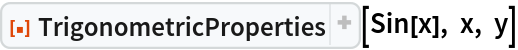Out=Return a specific trigonometric property:

 In:=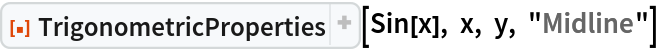Out=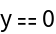Compute the amplitude:

 In:=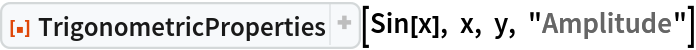Out=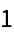### Possible Issues (4)

If a function is not periodic, the function returns unevaluated:

 In:=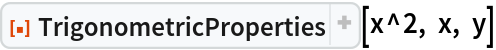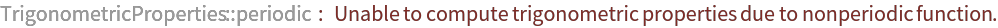Out=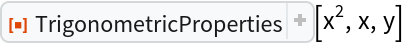If a function contains terms that are non-constant, non-trigonometric, the function returns unevaluated:

 In:=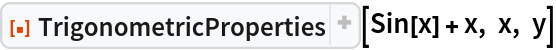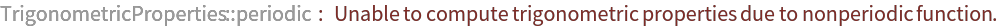Out=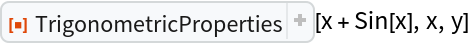TrigonometricProperties does not work with inverse trigonometric functions:

 In:=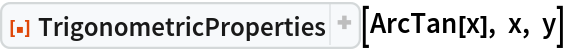Out=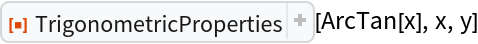Functions that do not reduce to a single trigonometric expression return unevaluated:

 In:=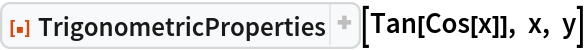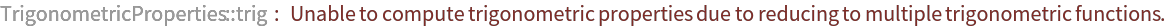Out=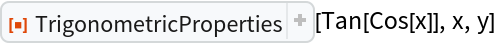## Publisher

Wolfram|Alpha Math Team

## Version History

• 2.0.0 – 23 March 2023
• 1.0.0 – 14 March 2022